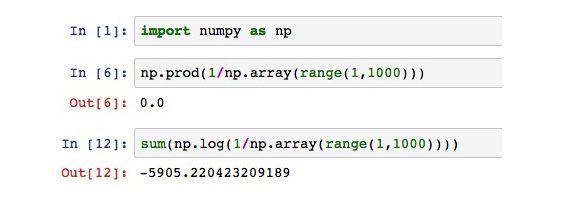Returns

The raw return may be referred to simply as the return, or alternatively, as the percentage return, linear return, or simple return. It is defined as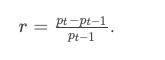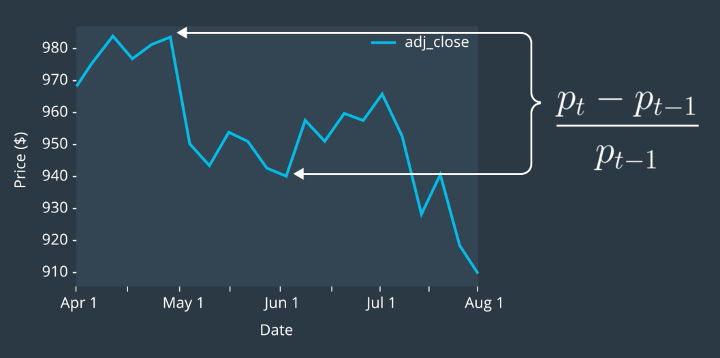练习

Calculate ReturnsUsing the formula $\frac{p{t} - p{t-1}}{p_{t-1}}$, let's apply it to some example prices. For this exercise, we'll calculate the returns for each day using the closing price data in close.

import pandas as pd

close = pd.DataFrame(
{
'ABC': [1, 5, 3, 6, 2],
'EFG': [12, 51, 43, 56, 22],
'XYZ': [35, 36, 36, 36, 37],},
pd.date_range('10/01/2018', periods=5, freq='D'))
close
ABC EFG XYZ
2018-10-01 1 12 35
2018-10-02 5 51 36
2018-10-03 3 43 36
2018-10-04 6 56 36
2018-10-05 2 22 37

Using the returns formula on the closing prices for the ticker "ABC" should give us [(5-1)/1, (3-5)/5, (6-3)/3, (2-6)/6] or [4, -0.4, 1, -0.66]. To calculate this for the whole DataFrame, we'll use the DataFrame.shift function.

This function allows us to shift the rows of data. For example, the following shifts the rows in close two days back.

close.shift(2)
ABC EFG XYZ
2018-10-01 NaN NaN NaN
2018-10-02 NaN NaN NaN
2018-10-03 1.0 12.0 35.0
2018-10-04 5.0 51.0 36.0
2018-10-05 3.0 43.0 36.0

The data for the row "2018-10-03" contains data that is two days in the past. You'll also notice the "NaN" values for "2018-10-01" and "2018-10-02". Since there's not data two days in the past for these dates, it returns a "NaN" value.

Use this function, you can also shift in the future using a negative number. Let's shift one day in the future.

close.shift(-1)
ABC EFG XYZ
2018-10-01 5.0 51.0 36.0
2018-10-02 3.0 43.0 36.0
2018-10-03 6.0 56.0 36.0
2018-10-04 2.0 22.0 37.0
2018-10-05 NaN NaN NaN

Quiz

Using what you know about the DataFrame.shift function, implement the function.

Once you successfully implemented the quiz, you can can continue to the next concept in the classroom.

import quiz_tests

def calculate_returns(close):
"""
Compute returns for each ticker and date in close.

Parameters
----------
close : DataFrame
Close prices for each ticker and date

Returns
-------
returns : DataFrame
Returns for each ticker and date
"""
# TODO: Implement Function

return (close - close.shift(1))/close.shift(1)

quiz_tests.test_calculate_returns(calculate_returns)
Tests Passed

Log Returns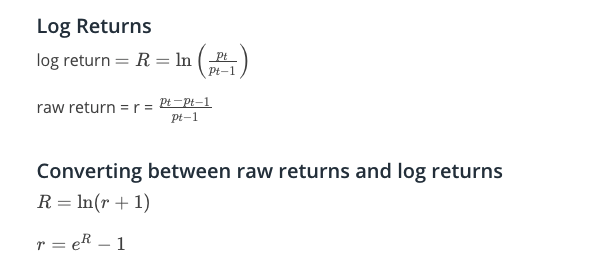Quiz

Log Returns and Compounding
Log returns can be convenient for calculations that involve compounding. To explain this idea, let's first discuss compounding.

If you invest $100 in an asset, and the rate of return is 4% per year, and the amount of interest accrued is only calculated after the money has sat in the account for 1 year, how much money do you have after 1 year? 如果您在一项资产上投资100美元，并且每年的回报率为4％，并且应计利息金额仅在资金在账户中存入1年后计算，那么1年后您将获得多少资金？ A：102 B：108 C：104 D：116 答案选C （2）Compounding 复利 he idea of compounding is a simple one. Say you put$100 in a bank account that earns 4% interest per year, compounded annually. After 1 year, you have

$100 +$100*0.04 = $104. The next year, you have,$104 + $104*0.04 =$108.16,

so you earned another $4 of interest on the original$100 but also 16 cents on the $4 of interest earned last year. Compounding is the process by which an asset’s earnings are reinvested to generate additional earnings, that is to say, earning interest on interest. 复利是指资产收益再投资以产生额外收益的过程，也就是说，赚取利息利息。 习题： Let's say you invest$100 in an asset. The annual rate of interest is reported at 4%, but the asset's seller has explained that what this means is that 2% is gained over 6 months, and then interest is calculated on the investment and it's reinvested, and then the process is repeated after the next 6 months. How much money will you have at the end of 1 year?

• A：104.04
• B：104.00
• C：102.00
• D：102.04

Rates of Compounding

A statement by a bank that the interest rate on one-year deposits is 4% per year sounds straightforward and unambiguous. In fact, its precise meaning depends on the way the interest rate is measured.

For an interest rate statement to be clear, the magnitude and time dependence of the rate of interest, as well as the frequency of compounding, must be clearly stated.

If the interest rate is measured with annual compounding, the bank’s statement that the interest rate is 4% means that $100 grows to \$100\times(1 + .04) = \$104$100×(1+.04)=$104 at the end of 1 year. When the interest rate is measured with semiannual compounding, it means that 2% is earned every 6 months, with the interest being reinvested. In this case,$100 grows to \$100\times(1 + .04/2)\times(1 + .04/2) = \$100\times(1 + .04/2)^2 = \$104.04$100×(1+.04/2)×(1+.04/2)=$100×(1+.04/2) 2 =$104.04, at the end of 1 year.

When the interest rate is measured with quarterly compounding, the bank’s statement means that 1% is earned every 3 months, with the interest being reinvested. The $100 then grows to \$100\times(1 + .04/4)^4 = \$104.06$100×(1+.04/4) 4 =$104.06 at the end of 1 year. Continuous Compounding Let's compare the amount of money accumulated after 1 year with the same annual rate of interest of 4%, but different rates of compounding: 让我们比较一年后积累的金额与相同的年利率4％，但不同的复利率：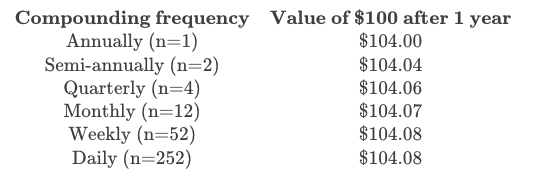Looking at the table, you can see that with more frequent compounding, the value at 1 year increases but then seems to level off. If you assumed that the benefit of compounding more and more frequently had a limit, you would be right! How do we calculate this limit? Well, first we write down the formula for compounding, 看一下表，你可以看到，随着更频繁的复合，1年的价值增加，但似乎趋于平稳。如果你认为复合的好处越来越频繁有限，你就是对的！我们如何计算这个限制？好吧，首先我们写下复合的公式，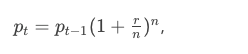and then we notice that what we'd like to do is make n bigger and bigger. We want the limit as n goes to infinity. Well, it turns out that this limit is: 然后我们注意到我们想要做的就是让n更大更大。当n变为无穷大时，我们想要限制。好吧，事实证明这个限制是：Compounding infinitely often is called continuous compounding. So what does this mean? Well, it means that if you wanted to calculate how much money you’d have at the end of the year if you started with$100 and compounded continuously, but at a simple annual rate of 4%, you’d calculate:

You'll notice that the value after 1 year with continuous compounding is pretty close (it's the same if we round to two decimal places) to the value after 1 year with daily compounding.

Continuously Compounded Return

Now, say you were trying to reverse the previous calculation. Say you knew you had $104.08 at the end of the year, and$100 at the beginning of the year, and you wanted to calculate the rate of interest as if it had been compounded continuously. You would simply invert the formula. So you’d calculate:

$104.08/$100=e^r

Then you'd take the natural log of both sides, and then you'd have,

\ln(\$104.08/\$100) = r

So,

.04 = r.04=r

r is the continuously compounded annual return. So the continuously compounded annual return equals \ln(pt/p{t-1})ln(pt/pt−1 ). But what is this quantity? It’s just the log return! This is why you might hear log returns called continuously compounded returns.

Annualized Rate of Return(年化收益率）

We saw above how to calculate the annual rate of continuous compounding from the monthly rate of continuous compounding. If we just had a single monthly rate, but we assumed that the rates for all the months of the year were the same, we could extrapolate the monthly rate to an annual rate by multiplying by 12. This is called annualizing the rate of continuous compounding. Rates of return and other metrics are often converted to a common annual basis in order to make comparisons across contexts and instruments.

So, as you can see, the rate of continuous compounding is additive over time. Since, mathematically, the rate of continuous compounding is just the log return, this means that log returns are additive over time, and this can be very convenient. As another example,

logreturnforJanuary+logreturnforFebruary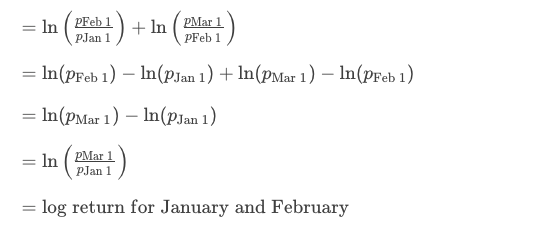Numerical Stability

Multiplication of many small numbers can result in the problem that the product is smaller than the smallest number representable in computer memory. Sometimes the computation will incorrectly yield the value 0. This is called arithmetic underflow. The use of logarithms can help with this, since it enables the representation of much smaller (and much larger) numbers. For example: